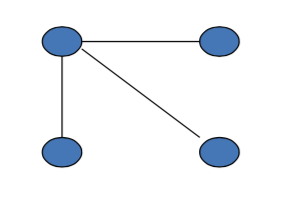## Efficient Networks in the Symmetric Connections Model¶

• low cost: $c < \delta - \delta^2$

• complete network is pairwise stable
• medium/low cost: $\delta - \delta^2 < c < \delta$

• star networks is pairwise stable
• others are also pairwise stable
• medium/high cost: $\delta < c < \delta + (n-2)\delta^2/2$

• star network is not pairwise stable (no loose ends)
• What does "no loose ends" mean? It means there's no individual that's going to want to connect to some other individual that doesn't bring them any indirect benefits. They'd rather sever that link because they're only getting a $\delta$ here and it's not worth it. So that certainly means that a star is not going to be worthwhile. The center is not going to be willing to take these other individuals who don't bring them anything besides themselves.
• nonempty pairwise stable networks are over-connected and may include too few agents
• $\delta < c$, basically what it means is "c" is bigger than $\delta$, so the cost the only reason you want to have a relationship is if it's bringing also some indirect benefits with it. In that case a star is still efficient but a star is not going to be pairwise stable.
• high cost: $\delta + (n - 2)\delta^2 / 2 < c$
• empty network is pairwise stable

## Inefficiency¶• payoff to center:

$3\delta - 3c$

not pairwise stable if

$\delta < c$

• Overall payoff:

$6\delta + 6\delta^2 - 6c$

Peripheral players gain indirect benefits

Center player does not account for them

## Example: Pairwise stable and inefficient¶

unique nonempty pairwise stable network architecture if

$\delta < c < (\delta + \delta^2 + \delta^3)(1 - \delta^2)$, n = 6Here's an example, you can play with this example if you like. Let delta be bigger than c and, in this case smaller than delta plus delta squared plus delta cubed, times 1 minus delta squared. six individuals, and there's a unique non-empty pairwise stable network architecture, which looks like this. So it doesn't look like a star, it, it actually looks like a ring or a circle. And the idea here is that each individual is willing to have these other relationships even though c is bigger than delta. So c is higher than delta, but that's because they get indirect benefits from having these. So by having these they, they get indirect benefits as well and that makes it worthwhile and it all hooks together. Nobody wants to add any extra links across because the value of, of adding that link is not worth the cost. It doesn't change the indirect relationships to anybody else but adds an extra cost. And it's not worth shortening that path from a delta cubed to a single one. So this, in this setting you can check is the unique pairwise stable nonempty network and we end up with something that is not a star.